Sept 2015

I will post seven new problems every weekend, one for each day of the week. The idea is that you work on your own during the week and I will post answers the following Friday at 7pm. Because this webpage went public only a few days ago, some kids have just discovered it and asked me whether they could have this weekend to finish the problems from the first two weeks. So I will wait till Sun Sept 27 to post the answers.  To those of you who have already submitted, thank you and keep practicing!  Answers for Sept 12-26 are posted under the week headers.

Information sheet: JerseySTEM would like to collect participant info.

1. From gmail.com login with your a jerseystem.net account.  If you don’t yet have one, please enter Username: guest@jerseystem.net, Password: mathisfun.
2. Click this information sheet and fill in info about yourself.  You will be issued a jerseystem.net account with the form firstname.lastname@jerseystem.net (like mine).

1. From gmail.com login with your a jerseystem.net account. If you don’t yet have one, please enter Username: guest@jerseystem.net, Password: mathisfun.
2. Click GoogleForm after the week header, e.g. Week of September 12-18, Week of September 19-25, to submit the weekly answers. Make sure you write your name on the form and submit your answers before I post mine on Friday at 7pm.

Prizes:  As encouragement, JerseySTEM is offering prizes to recognize those who have consistently answered questions correctly. The first round of prizes will be given out on Friday, Oct 23, 2015, to recognize high performers for the time period Sept 12 – Oct 16, 2015. Details to follow.

Week of Sept. 26 – Oct. 2: This week’s topic is about computing average. Click GoogleForm to submit this week’s answers.

Answers: D, D, C, E, A, D B

• Sept 26

Theresa’s parents have agreed to buy her tickets to see her favorite band if she spends an average of$10$ hours per week helping around the house for$6$ weeks. For the first$5$ weeks she helps around the house for$8$,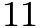$11$,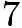$7$,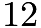$12$ and$10$ hours. How many hours must she work for the final week to earn the tickets?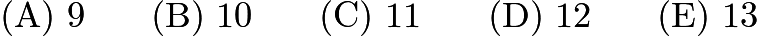$\mathrm{(A)}\ 9 \qquad\mathrm{(B)}\ 10 \qquad\mathrm{(C)}\ 11 \qquad\mathrm{(D)}\ 12 \qquad\mathrm{(E)}\ 13$

Source: AMC8 2007

• Sept 27

The average age of$5$ people in a room is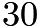$30$ years. An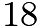$18$-year-old person leaves the room. What is the average age of the four remaining people?$\mathrm{(A)}\ 25 \qquad\mathrm{(B)}\ 26 \qquad\mathrm{(C)}\ 29 \qquad\mathrm{(D)}\ 33 \qquad\mathrm{(E)}\ 36$

Source: AMC8 2007

• Sept 28

The numbers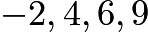$-2, 4, 6, 9$ and$12$ are rearranged according to these rules:

1. The largest isn’t first, but it is in one of the first three places.
2. The smallest isn’t last, but it is in one of the last three places.
3. The median isn’t first or last.

What is the average of the first and last numbers?$\textbf{(A)}\ 3.5 \qquad \textbf{(B)}\ 5 \qquad \textbf{(C)}\ 6.5 \qquad \textbf{(D)}\ 7.5 \qquad \textbf{(E)}\ 8$

Source: AMC8 2004

• Sept 29

Gage skated 1 hr 15 min each day for 5 days and 1 hr 30 min each day for 3 days. How long would he have to skate the ninth day in order to average 85 minutes of skating each day for the entire time?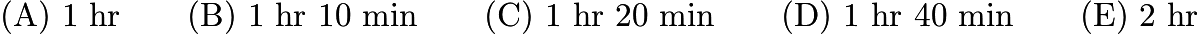$\text{(A)}\ \text{1 hr} \qquad \text{(B)}\ \text{1 hr 10 min} \qquad \text{(C)}\ \text{1 hr 20 min} \qquad \text{(D)}\ \text{1 hr 40 min} \qquad \text{(E)}\ \text{2 hr}$

Source: AMC8 2002

• Sept 30

Blake and Jenny each took four 100-point tests. Blake averaged 78 on the four tests. Jenny scored 10 points higher than Blake on the first test, 10 points lower than him on the second test, and 20 points higher on both the third and fourth tests. What is the difference between Jenny’s average and Blake’s average on these four tests?$\mathrm{(A)}\ 10 \qquad\mathrm{(B)}\ 15 \qquad\mathrm{(C)}\ 20 \qquad\mathrm{(D)}\ 25 \qquad\mathrm{(E)}\ 40$

Source: AMC8 2003

• Oct 1

The average of the five numbers in a list is$54$. The average of the first two numbers is$48$. What is the average of the last three numbers?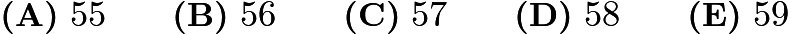$\textbf{(A)}\ 55\qquad\textbf{(B)}\ 56\qquad\textbf{(C)}\ 57\qquad\textbf{(D)}\ 58\qquad\textbf{(E)}\ 59$

Source: AMC8 2004

• Oct 2

There is a list of seven numbers. The average of the first four numbers is 5, and the average of the last four numbers is 8. If the average of all seven numbers is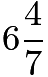$6\frac{4}{7}$, then the number common to both sets of four numbers is$\text{(A)}\ 5\frac{3}{7} \qquad \text{(B)}\ 6 \qquad \text{(C)}\ 6\frac{4}{7} \qquad \text{(D)}\ 7 \qquad \text{(E)}\ 7\frac{3}{7}$

Source: AMC8 2000

Week of September 19-25: This week’s topic is geometry. Click GoogleForm to submit this week’s answers.

Answers: A, C, C, D, C, B, C

• Sept 19

Figure$ABCD$ is a square. Inside this square three smaller squares are drawn with the side lengths as labeled. The area of the shaded L-shaped region is$[asy] pair A,B,C,D; A = (5,5); B = (5,0); C = (0,0); D = (0,5); fill((0,0)--(0,4)--(1,4)--(1,1)--(4,1)--(4,0)--cycle,gray); draw(A--B--C--D--cycle); draw((4,0)--(4,4)--(0,4)); draw((1,5)--(1,1)--(5,1)); label($$\text{(A)}\ 7 \qquad \text{(B)}\ 10 \qquad \text{(C)}\ 12.5 \qquad \text{(D)}\ 14 \qquad \text{(E)}\ 15$

Source: AMC8 2000

• Sept 20

What is the degree measure of the smaller angle formed by the hands of a clock at 10 o’clock?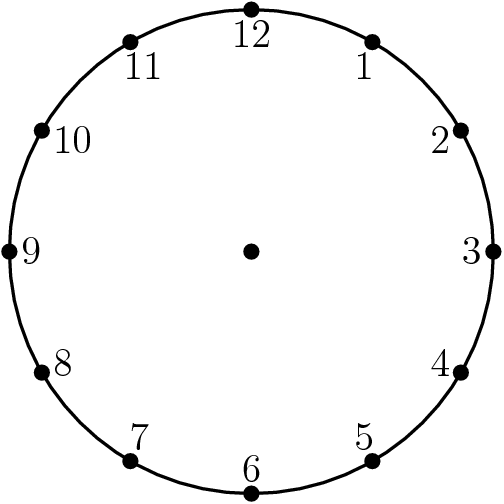$[asy] draw(circle((0,0),2)); dot((0,0)); for(int i = 0; i < 12; ++i) { dot(2*dir(30*i)); } label($$\text{(A)}\ 30 \qquad \text{(B)}\ 45 \qquad \text{(C)}\ 60 \qquad \text{(D)}\ 75 \qquad \text{(E)}\ 90$

Source: AMC8 1999

• Sept 21

In triangle$CAT$, we have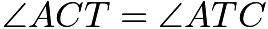$\angle ACT = \angle ATC$ and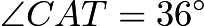$\angle CAT = 36^\circ$. If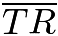$\overline{TR}$ bisects$\angle ATC$, then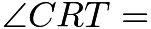$\angle CRT =$$[asy] pair A,C,T,R; C = (0,0); T = (2,0); A = (1,sqrt(5+sqrt(20))); R = (3/2 - sqrt(5)/2,1.175570); draw(C--A--T--cycle); draw(T--R); label($$\text{(A)}\ 36^\circ \qquad \text{(B)}\ 54^\circ \qquad \text{(C)}\ 72^\circ \qquad \text{(D)}\ 90^\circ \qquad \text{(E)}\ 108^\circ$

Source: AMC8 2000

• Sept 22

In trapezoid$ABCD$, the sides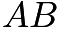$AB$ and$CD$ are equal. The perimeter of$ABCD$ is$[asy] draw((0,0)--(4,3)--(12,3)--(16,0)--cycle); draw((4,3)--(4,0),dashed); draw((3.2,0)--(3.2,.8)--(4,.8)); label($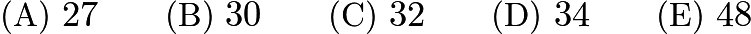$\text{(A)}\ 27 \qquad \text{(B)}\ 30 \qquad \text{(C)}\ 32 \qquad \text{(D)}\ 34 \qquad \text{(E)}\ 48$

Source: AMC8 1999

• Sept 23

Triangles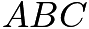$ABC$$ADE$, and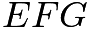$EFG$ are all equilateral. Points$D$ and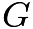$G$ are midpoints of$\overline{AC}$ and$\overline{AE}$, respectively. If$AB = 4$, what is the perimeter of figure$ABCDEFG$?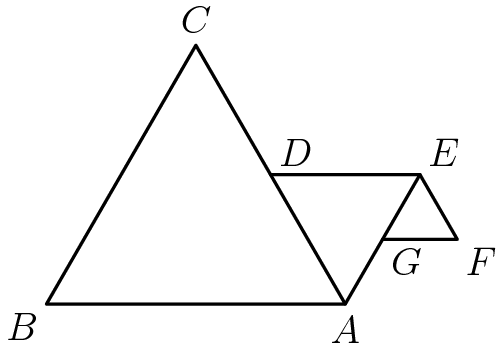$[asy] pair A,B,C,D,EE,F,G; A = (4,0); B = (0,0); C = (2,2*sqrt(3)); D = (3,sqrt(3)); EE = (5,sqrt(3)); F = (5.5,sqrt(3)/2); G = (4.5,sqrt(3)/2); draw(A--B--C--cycle); draw(D--EE--A); draw(EE--F--G); label($$\text{(A)}\ 12 \qquad \text{(B)}\ 13 \qquad \text{(C)}\ 15 \qquad \text{(D)}\ 18 \qquad \text{(E)}\ 21$

Source: AMC 2000

• Sept 24

Given the areas of the three squares in the figure, what is the area of the interior triangle?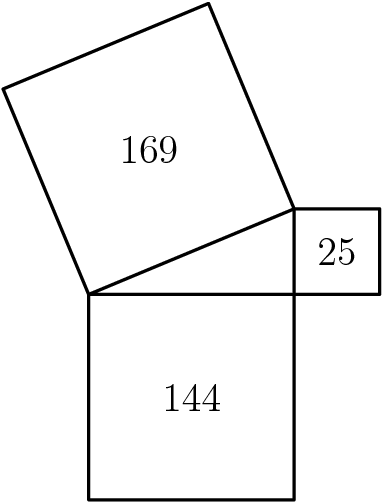$[asy] draw((0,0)--(-5,12)--(7,17)--(12,5)--(17,5)--(17,0)--(12,0)--(12,-12)--(0,-12)--(0,0)--(12,5)--(12,0)--cycle,linewidth(1)); label($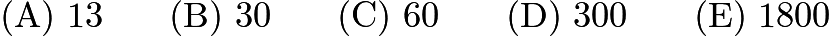$\mathrm{(A)}\ 13 \qquad\mathrm{(B)}\ 30 \qquad\mathrm{(C)}\ 60 \qquad\mathrm{(D)}\ 300 \qquad\mathrm{(E)}\ 1800$

Source: AMC8 2003

• Sept 25

The following figures are composed of squares and circles. Which figure has a shaded region with largest area?$[asy]/* AMC8 2003 #22 Problem */ size(3inch, 2inch); unitsize(1cm); pen outline = black+linewidth(1); filldraw((0,0)--(2,0)--(2,2)--(0,2)--cycle, mediumgrey, outline); filldraw(shift(3,0)*((0,0)--(2,0)--(2,2)--(0,2)--cycle), mediumgrey, outline); filldraw(Circle((7,1), 1), mediumgrey, black+linewidth(1)); filldraw(Circle((1,1), 1), white, outline); filldraw(Circle((3.5,.5), .5), white, outline); filldraw(Circle((4.5,.5), .5), white, outline); filldraw(Circle((3.5,1.5), .5), white, outline); filldraw(Circle((4.5,1.5), .5), white, outline); filldraw((6.3,.3)--(7.7,.3)--(7.7,1.7)--(6.3,1.7)--cycle, white, outline); label($$\textbf{(A)}\ \text{A only}\qquad\textbf{(B)}\ \text{B only}\qquad\textbf{(C)}\ \text{C only}\qquad\textbf{(D)}\ \text{both A and B}\qquad\textbf{(E)}\ \text{all are equal}$

Source: AMC8 2003

Week of September 12-18: This is the first week, so let’s start easy with some simple calculation problems. These happen to be initial questions in many of the past tests. Click GoogleForm to submit this week’s answers.

Answers: B, A, D, B, C, D, E

• Sept 12

For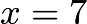$x=7$, which of the following is the smallest?$\text{(A)}\ \dfrac{6}{x} \qquad \text{(B)}\ \dfrac{6}{x+1} \qquad \text{(C)}\ \dfrac{6}{x-1} \qquad \text{(D)}\ \dfrac{x}{6} \qquad \text{(E)}\ \dfrac{x+1}{6}$

Source: AJHSME 1998 (AJSHME is the old name for AMC8)

• Sept 13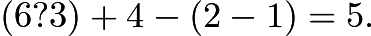$(6?3) + 4 - (2 - 1) = 5.$ To make this statement true, the question mark between the 6 and the 3 should be replaced by$\text{(A)} \div \qquad \text{(B)}\ \times \qquad \text{(C)} + \qquad \text{(D)}\ - \qquad \text{(E)}\ \text{None of these}$

Source: AMC8 1999

• Sept 14

Which triplet of numbers has a sum NOT equal to 1?$\text{(A)}\ (1/2,1/3,1/6) \qquad \text{(B)}\ (2,-2,1) \qquad \text{(C)}\ (0.1,0.3,0.6) \qquad \text{(D)}\ (1.1,-2.1,1.0) \qquad \text{(E)}\ (-3/2,-5/2,5)$

Source: AMC8 1999

• Sept 15

Aunt Anna is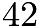$42$ years old. Caitlin is$5$ years younger than Brianna, and Brianna is half as old as Aunt Anna. How old is Caitlin?$\text{(A)}\ 15 \qquad \text{(B)}\ 16 \qquad \text{(C)}\ 17 \qquad \text{(D)}\ 21 \qquad \text{(E)}\ 37$

Source: AMC8 2000

• Sept 16

Each principal of Lincoln High School serves exactly one$3$-year term. What is the maximum number of principals this school could have during an$8$-year period?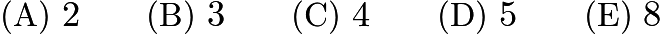$\text{(A)}\ 2 \qquad \text{(B)}\ 3 \qquad \text{(C)}\ 4 \qquad \text{(D)}\ 5 \qquad \text{(E)}\ 8$

Source: AMC8 2000

• Sept 17

Casey’s shop class is making a golf trophy. He has to paint 300 dimples on a golf ball. If it takes him 2 seconds to paint one dimple, how many minutes will he need to do his job?$\text{(A)}\ 4 \qquad \text{(B)}\ 6 \qquad \text{(C)}\ 8 \qquad \text{(D)}\ 10 \qquad \text{(E)}\ 12$

Source: AMC8 2001

• Sept 18

Granny Smith has $63. Elberta has$2 more than Anjou and Anjou has one-third as much as Granny Smith. How many dollars does Elberta have?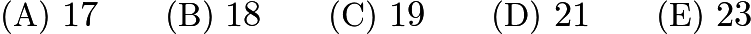$\text{(A)}\ 17 \qquad \text{(B)}\ 18 \qquad \text{(C)}\ 19 \qquad \text{(D)}\ 21 \qquad \text{(E)}\ 23$

Source: AMC8 2001